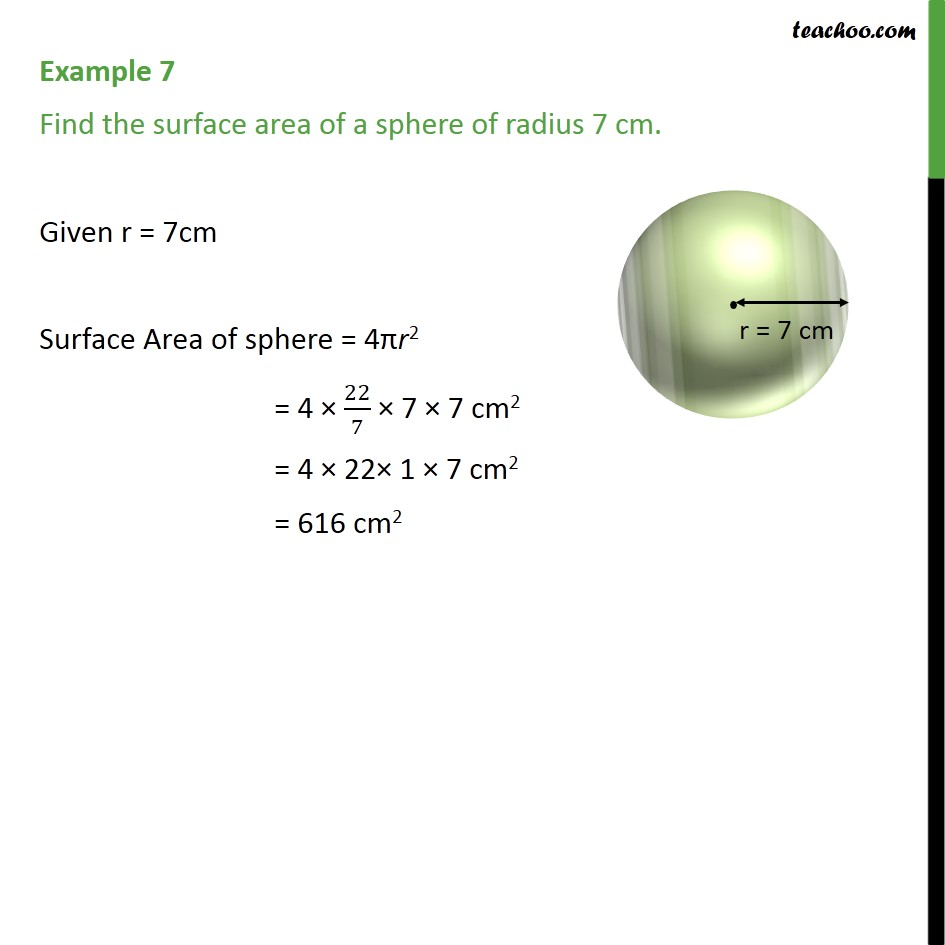1. Chapter 13 Class 9 Surface Areas and Volumes (Term 2)
2. Serial order wise
3. Examples

Transcript

Example 7 Find the surface area of a sphere of radius 7 cm. Given r = 7cm Surface Area of sphere = 4πr2 = 4 × 22/7 × 7 × 7 cm2 = 4 × 22× 1 × 7 cm2 = 616 cm2